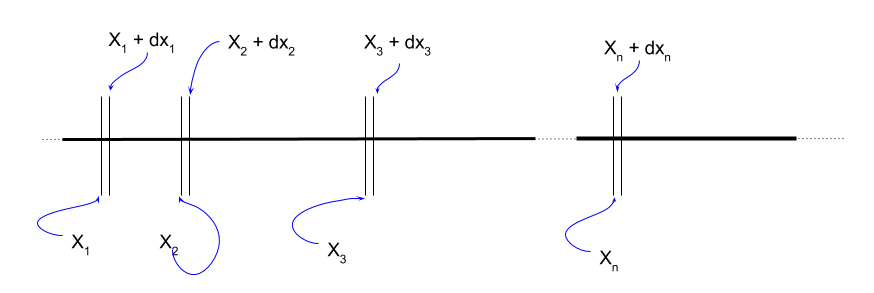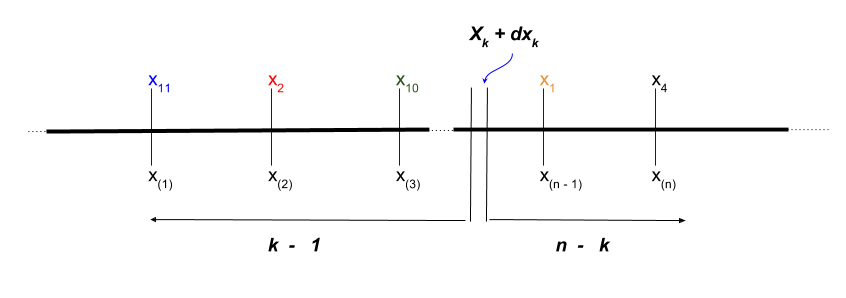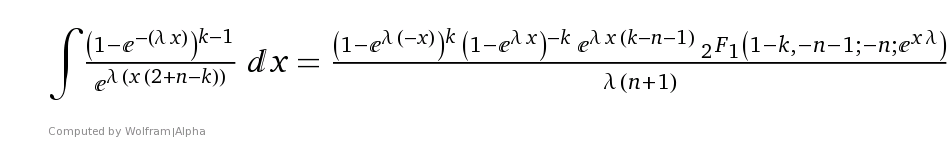### MOTIVATION FOR k ORDER STATISTICS:

The motivation stems from this question on CV.

A website receives $$1\, \text{order}\,/3 \, \text{min}$$. The time until the next order follows an exponential distribution with a $$\lambda$$ rate parameter of $$3 \, \text{min}$$. Knowing that there were $$10$$ clients placing orders online from that site last night we want to calculate the expected waiting time for the first and last orders placed after 5pm (night-time period = time $$0$$). And we want to find out the time of the $$k$$-th order.

Every order placed cames at a time. This time is the random variable. Since there are 10 orders, we have $$X_1, X_2, X_3,...X_{10}$$. To make the derivation more generalized, we can consider $$X_n$$ as the last entry. They are independent of each other, and they are governed by the pdf $$\lambda\,e^{-\lambda\,x}$$, and cdf $$1 \,-\, e^-{-\lambda\,x}$$, where $$x$$ stands for time. These will be referred as the common density and probability functions, because they are shared by all the variables.

The point now is to describe a different variable: the order of the observations. So if we imagine that the callers carry a bib number from $$1$$ to $$10$$ that links to the times they place their orders, client $$X_1$$ may end up being the third one to place the order, when ordering the times of the $$10$$ clients, in which case the new variable will be denoted as $$X_{(3)}$$. Notice the small parentheses.

The cdf of this new variable for the last order, $$n=10$$ will be:$$F_{x_{(n)}}(x) = \Pr(X_{n}\leq x) = \Pr\left(\text{all}\,X_i \leq x\right) = \displaystyle \prod_{k=1}^n \Pr(X_n \leq x) = F(x)^n$$, since they are independent and identically ditributed.

In the case of the exponential distribution: $$\left (1 - e^{-\lambda\,x \,}\right)^n.$$

On the other hand the pdf is:

$$\frac{d}{dx}\,F_{x_{(n)}}(x) = n\,(F(x))^{n-1}\, f(x) = n\,\left (1 - e^{-\lambda\,x \,}\right)^{n-1}\,\lambda\,e^{-\lambda x}.$$

The pdf and cdf for the first order $$(n=1)$$ entered on the website are:

\begin{align} F_{x_{(1)}}(x) &= P(\text{min}\, X_i \leq x)\\[2ex] &= 1 - P(X_{(1)} \geq x)\\[2ex] &= 1 - (1 - F(x))^n\\[2ex] &= 1 - (1 - (1 - e^{-\lambda x}))^n\\[2ex] &= 1 - e^{\,-\lambda x n}. \end{align}

The pdf for the minimum will be:

\begin{align} f_{X_{(1)}}(x)&=\large \frac{d}{dx}\,F_{x_{(1)}}(x)\\[2ex] &= - n \left(1 - F(x)\right)^{n-1}\,(-f(x))\\[2ex] &= n \left(1 - F(x)\right)^{n-1}\,(f(x))\\[2ex] &= n(1 - (1 - e^{-\lambda x}))^{n-1}\, \lambda\,e^{-\lambda x}\\[2ex] &= n(e^{-\lambda x (n-1)})\, \lambda\,e^{-\lambda x}\\[2ex] &= n\, \lambda \, e^{-\lambda x n}. \end{align}

What is the joint pdf for all the order statistics:For the actual observations evaluated at the the observation points:

\begin{align} \Pr(X_1 \in [x_1, x_1 + dx_1], X_2 \in [x_2, x_2 + dx_2], \cdots, X_n \in [x_n, x_n + dx_n]) &= \displaystyle \prod_{k=1}^n \, P(X_k \in [X_k, x_k + dx_k])\\[2ex] &= \displaystyle \prod_{k=1}^n \, f(x_k)\,dx_k\\[2ex] &=f(x_1)\,f(x_2)\,\cdots,f(x_n)\,dx_1\,dx_2,\cdots,\,dx_n. \end{align}

The first part is the joint pdf for $$\large f_{x_1,x_2,\cdots,x_n}$$ evaluated at $$\large x_1, x_2,\cdots, x_n$$, or

$$\large f_{x_1,x_2,\cdots,x_n\,(x_1, x_2,\cdots, x_n)}\large \,dx_1\,dx_2\,dx_3,\cdots\,dx_n$$ given the independence of the marginals.

Now, for the order statistics we need to see the indifference to the actual relationship between subject and order:

\begin{align} f_{x_{(1)},x_{(2)},x_{(3)}\cdots,x_{(n)}\,(x_1, x_2,\cdots, x_n)}\,dx_1\,dx_2\,dx_3,\cdots\,dx_n &=\Pr\left(X_{(1)} \in [x_1, x_1 + dx_1], X_{(2)} \in [x_2, x_2 + dx_2], \cdots, X_{(n)} \in [x_n, x_n + dx_n]\right)\\[2ex] &= n! f(x_1)\,f(x_2)\,\cdots,f(x_n)\large \,dx_1\,dx_2\,dx_3,\cdots\,dx_n \end{align}

And the pdf is $$\large n!\, f(x_1)\,f(x_2)\,\cdots,f(x_n).$$

For the marginal pdf of the $$\large K$$-th order statistic:We look at the probability of the observed value to be in the $$k$$ small interval. There are $$n$$ choices for the one value in the small interval ($$X_{(k)}$$) with probability $$f(x)\,dx$$. There are $$k - 1$$ to the left; and $$n - k$$ to the right. So we choose the ones to the left with the combination below, and we look at the probability of being to the left of $$x$$: $$\large F(x)^{k-1}\,(1-F(x))^{n-k})$$ in a binomial:

$f_{x{(k)}}(x)\,dx = n\,\binom{n-1}{k-1} (f(x)\,dx) \,F(x)^{k-1}\,\left(1-F(x)\right)^{n-k}$

Simplifying,

$f_{x{(k)}}(x) = n\,\binom{n-1}{ k-1} \, \,F(x)^{k-1}\,\left(1-F(x)\right)^{n-k}\, f(x)$

In the case of the exponential distribution:

\begin{align} f_{x{(k)}} &= n\,\binom{n-1}{ k-1} \, \,\left(1 - e^{\,-\lambda x}\right)^{k-1}\,\left(1-(1 - e^{\,-\lambda x})\right)^{n-k}\, f(x)\\[2ex] &=n\,\binom{n-1}{ k-1} \, \,(1 - e^{\,-\lambda x})^{k-1}\,( e^{\,-\lambda x})^{n-k}\, f(x)\\[2ex] &=n\,\binom{n-1}{k-1} \, \,(1 - e^{\,-\lambda x})^{k-1}\,( e^{\,-\lambda x})^{n-k}\, \lambda e^{\,-\lambda x}\\[2ex] &=\lambda \, n\,\binom{n-1}{k-1} \, \,(1 - e^{\,-\lambda x})^{k-1}\,( e^{\,-\lambda x})^{n-k}\, e^{\,-\lambda x}\\[2ex] &=\lambda \, n\,\binom{n-1}{ k-1} \, \,(1 - e^{\,-\lambda x})^{k-1}\,e^{\,-\lambda x(2 +n - k)} \end{align}

Integrating:And $$_2F_1$$(a,b; c; x) is the hypergeometric function.